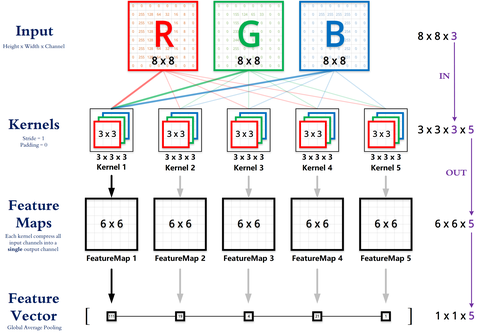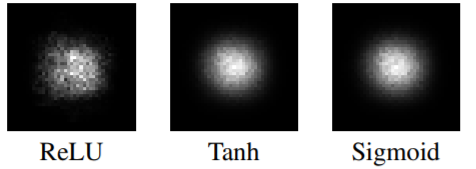1. 卷积层的作用？
2. 池化层的作用？
3. 卷积层的卷积核的大小选取？
4. 池化层的参数设定？

1. 全链接层的作用？
2. 1*1的卷积核的作用？
3. 卷积神经网络为什么效果这么好？

## 卷积层的作用？

1. 提取图像的特征，并且卷积核的权重是可以学习的，由此可以猜测，在高层神经网络中，卷积操作能突破传统滤波器的限制，根据目标函数提取出想要的特征

2.

“局部感知，参数共享”的特点大大降低了网络参数，保证了网络的稀疏性，防止过拟合

## 池化层的作用？

1. 特征不变性（feature invariant）

2. 特征降维

3. 在一定程度上能防止过拟合的发生

## 卷积层的卷积核的大小的选取？

// 关于感受野这个东西我知道这里写的很不清楚，所以在更新二中又写了一些，希望能更详细一点

1. 增强网络容量和模型复杂度
2. 减少卷积参数个数

1. 可充分利用和处理输入图像（或输入数据）的边缘信息（如下图所示）
2. 搭配合适的卷积层参数可保持输出与输入同等大小，而避免随着网络深度增加，输入大小的急剧减小## 全链接层的作用？

1. 全链接层相当于一个分类器

2.

3.

##卷积核的作用？

1. 降维或者升维，也可以当成“实现跨通道的交互和信息整合”
2. 以较少的参数的代价加深加宽了深度学习网络的层数，加入了更多的非线性信息

Michael Yuan：CNN中卷积层的计算细节zhuanlan.zhihu.com## 卷积神经网络为什么如此有效？

Yann LeCun在IJCAI 2018上的演讲

Yann LeCun 随后就对深度卷积网络的表征方式做了介绍

-----------------------------

yizt/numpy_neural_networkgithub.comimport numpy as np

def conv_forward( z, K, b, padding=(1,1), strides=(2,2) ):

'''
mutli channel conv forward
:param z: input matrix, shape is (N, C, H, W), N is batch_size, C is channels
:param K: kernel, shape is (C, D, k1, k2), C is input channel numbers, D is output channel numbers
:param b: bias, shape is (D,)
:param strides: strides
:return: the result of conv
'''

N, _, height, width = padding_z.shape
C, D, k1, k2 = K.shape

assert (height - k1) % strides == 0
assert (width - k2) % strides == 0

conv_z = np.zeros( (N, D, 1 + (height - k1) // strides, 1 + (width - k2) // strides) )

for n in np.arange(N):
for d in np.arange(D):
for h in np.arange(height - k1 + 1)[::strides]:
for w in np.arange(width - k2 + 1)[::strides]:

conv_z[n, d, h // strides, w // strides] = np.sum( padding_z[n, :, h: h + k1, w: w + k2] * K[:, d] ) + b[d]
return conv_z

z = np.ones( (1, 1, 5, 5) )
K = np.ones( (1, 1, 3, 3) ) - 0.5
b = np.ones( (1,) )

print conv_forward( z, K, b )表示第层，表示第层的感受野，表示第层的卷积或者池化的表示第层卷积或者池化的mileistone：关于感受野的总结zhuanlan.zhihu.com